Thursday 04th June 2020
 CBSE Guess > Papers > Question Papers > Class XII > 2005 > Chemistry > Outside Delhi Set-II CHEMISTRY 2005 (Set-II Outside Delhi)
Except for the following questions, all the remaining questions have bees asked In Set I. Q. 19. (a) Write one chemical reaction each to show that (i) Cone H2SO4 can an oxidizing agent. (ii) In (III) is more stable than In (I) in aqueous solutions. (b) Write the structural formula for either XeO3 or PCI5(s). 3 Q. 21. When the Isotopes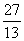AI,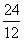Mg and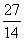Si were separately subjected to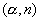reactions, three different nuclei were formed. Each of these new nuclei further emitted a positron giving a stable final nucleus. Write the related nuclear reaction equations. 3 Q. 22. (a) Write the structural formula for 4-methy I-pent-3-en-2-one. (b) To illustrate the following write one chemical reaction in each case: (i) Cannizzaro's reaction (ii) Wacker's process for converting ethylene to ethanal 3 Q. 23. Describe the following with suitable example: 3 (i) Antioxidants (ii) Analgesics (iii) Hybrid propellants Q. 25. (a) The outer electronic configurations of two members of the lanthanoid series are as follows: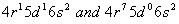What are their atomic numbers? Predict the oxidation states exhibited by these elements in their compounds. (b) Assign reason for each of the following statements: (i) The largest number of oxidation states are exhibited by the elements in the mldd1 e first row of the transition elements. (ii) The atomic radii decrease in size with the Increasing atomic number in the lanthanoid series. 5 Or  (a) Explain giving reasons: (i) Transition elements and their compounds exhibit paramagnetic behaviour. (ii) Transition elements and their compounds are found to be good catalysts. (iii) The enthalpies of atomization of the transition elements are high. (b) Compare the chemistry of actinoids with that of the transition elements lanthanoids in reference to (i) atomic and ionic sizes (ii) oxidation states Q. 26. (a) Explain how the concept of coupling of reactions Is useful in carrying out a non-spontaneous reaction. (b) Calculate the standard Gibbs energy change, Ar G°, for the following re action at 298 K using standard Gibbs energies of formation (Af G°) provided to you. 5 CaCO3 (s) +2H+ (aq) --> Ca2+ (aq) + H2 O (l) + CO2 (g) CaCO3 (s) =- 1128.8 ; H2O (f) =- 237.13 Ca2+ (aq) =-553.58 ; H+ (aq) =0, CO2 (g) =- 394.36 Or (a) Define the following terms: (i) State functions (ii) Gibbs energy change (b) Proceeding from the enthalpy of formation of gaseous carbon dioxide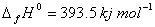and the thermochemical equation C(graphite)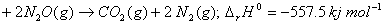calcuIate the enthalpy of formation of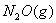. English 2005 Question Papers Class XII Delhi Outside Delhi Set I Set I Set II Set II Set III Set III CBSE 2005 Question Papers Class XII